# Blackboard Shots with Prefix "17-1750"

17-1750 is 2017 MAT 1750 - Shameless Mathematica.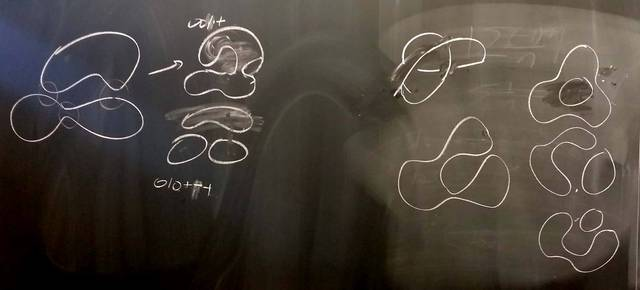171127-111331: KH: A 2-crossing example (4).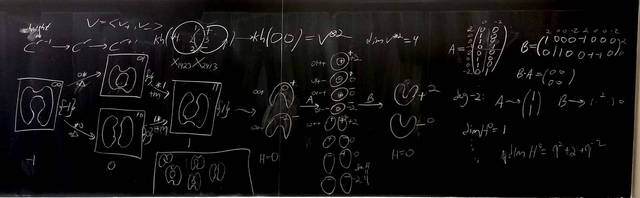171127-111330: KH: A 2-crossing example (3).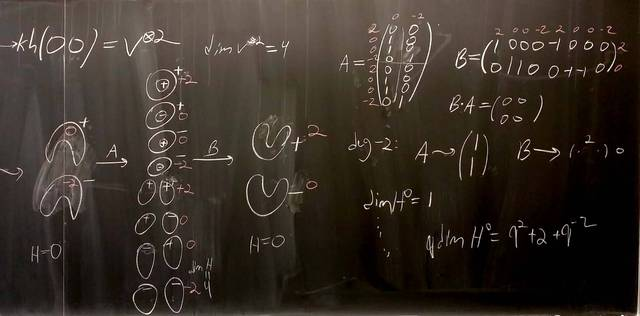171127-111329: KH: A 2-crossing example (2).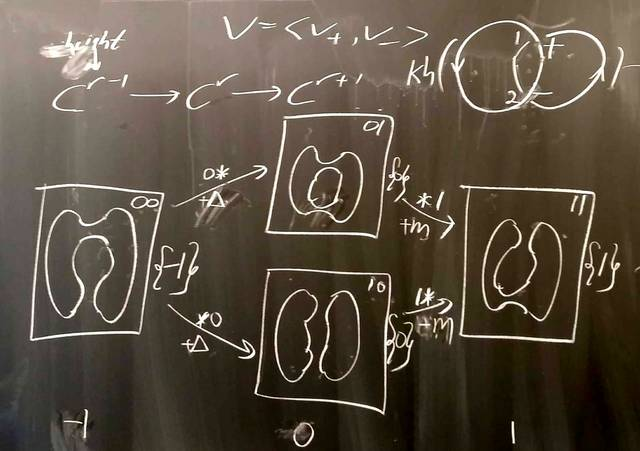171127-111328: KH: A 2-crossing example.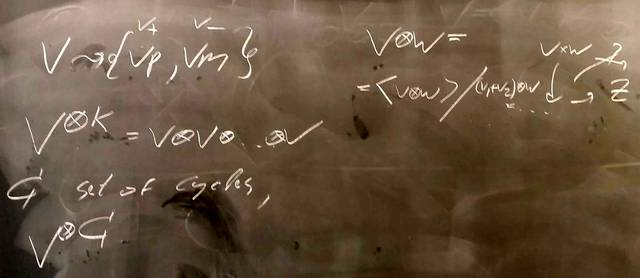171124-142441: Khovanov homology, in theory (5).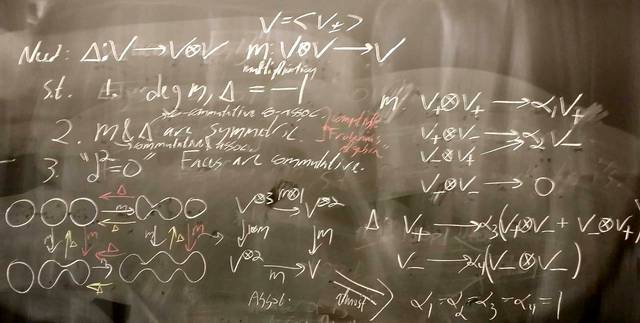171124-142440: Khovanov homology, in theory (4).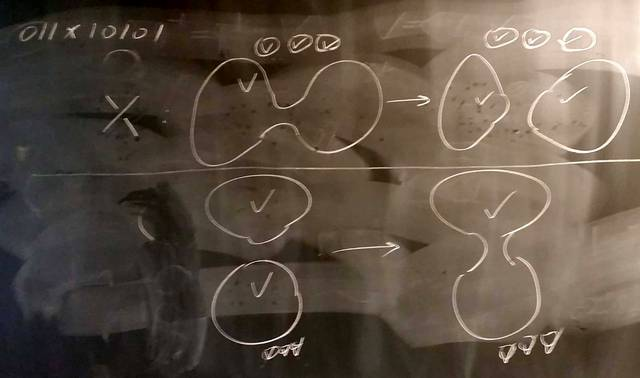171124-142439: Khovanov homology, in theory (3).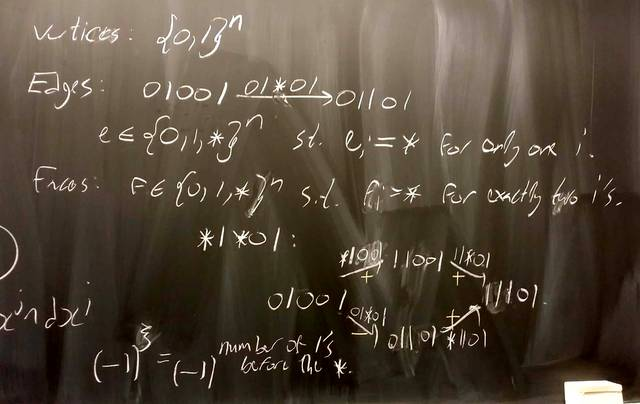171124-142438: Khovanov homology, in theory (2).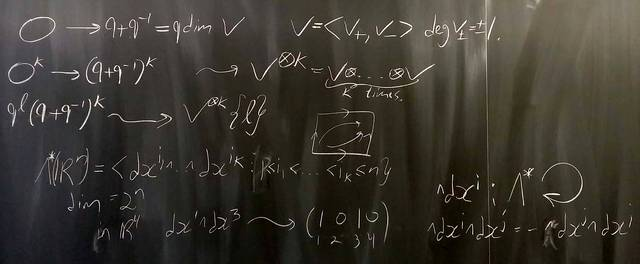171124-142437: Khovanov homology, in theory.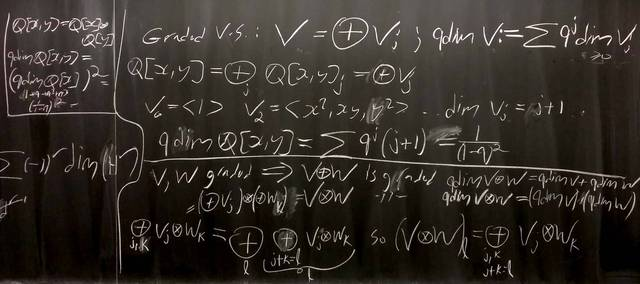171120-110737: Categorifying Laurent polynomials.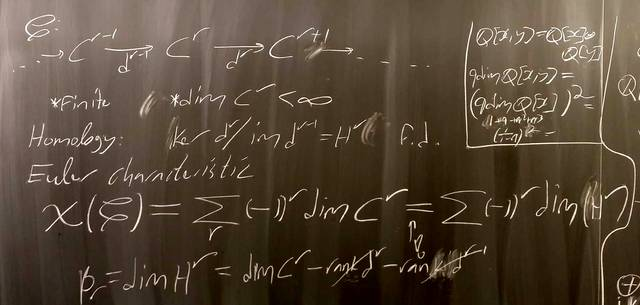171120-110736: Categorifying ${\mathbb Z}$.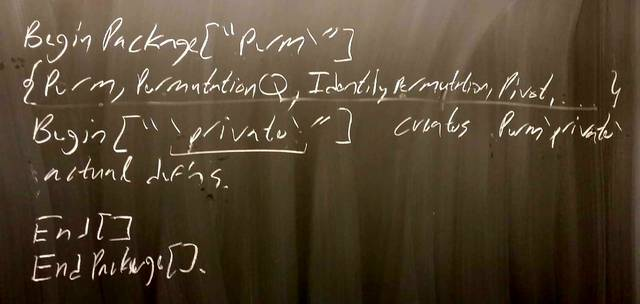171117-112505: The structure of packages.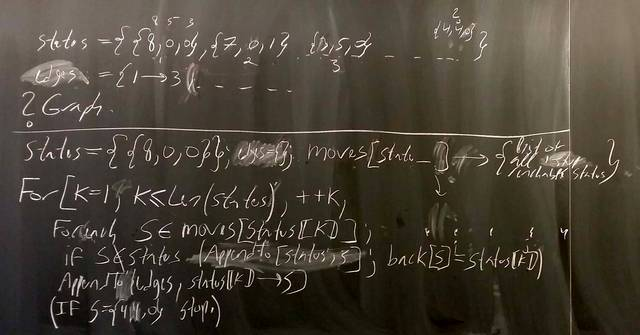171117-112504: The milk-jug problem.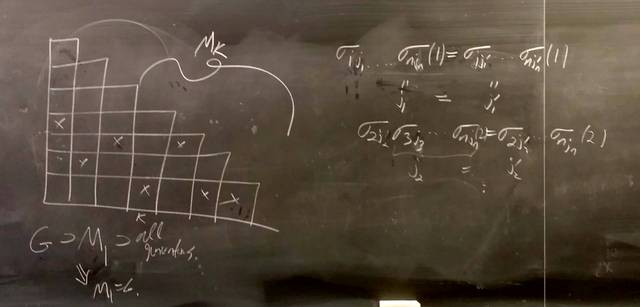171106-111254: Non-commutative Gaussian elimination (2).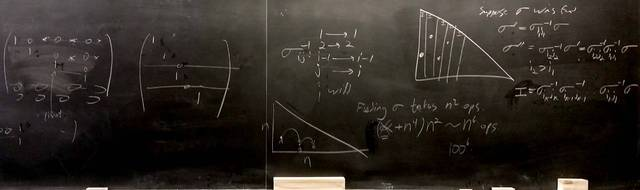171103-133255: Non-commutative Gaussian elimination.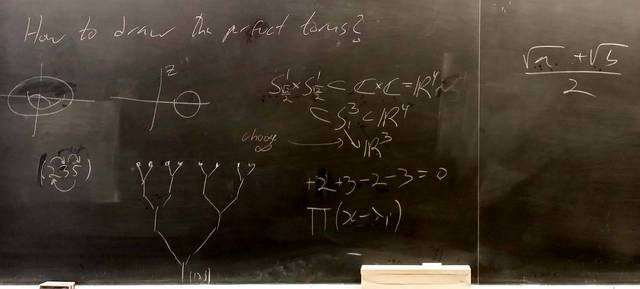171103-133254: Not solving the quintic.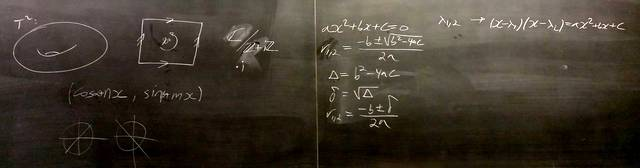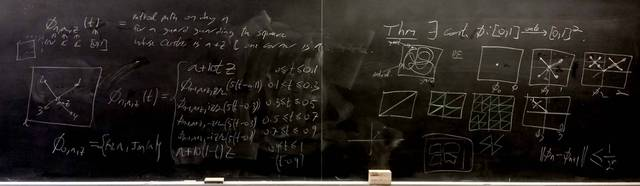171027-121227: The Peano Curve (2).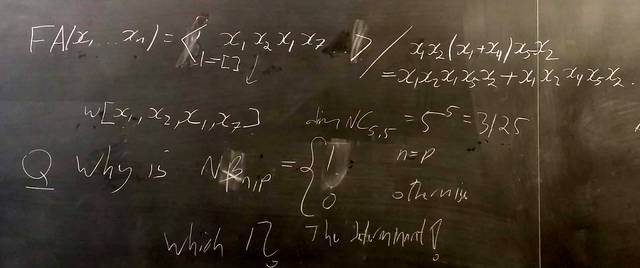171027-121225: Basic homology (7).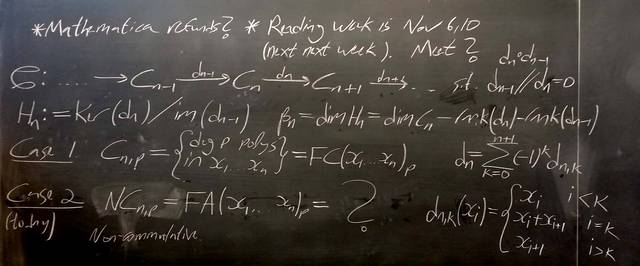171027-121223: Basic homology (6).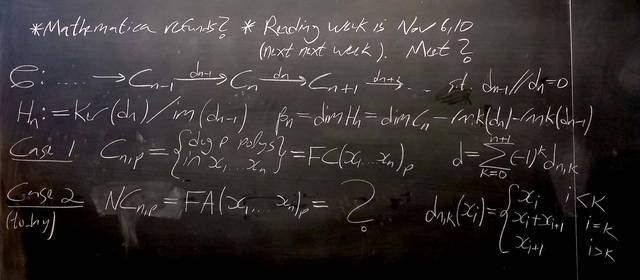171027-121222: Basic homology (5).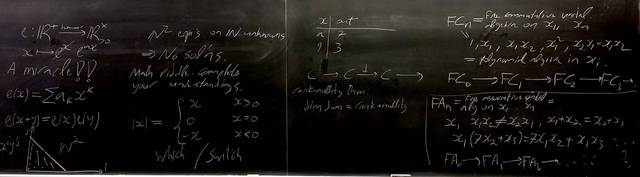171023-111128: Basic homology (4).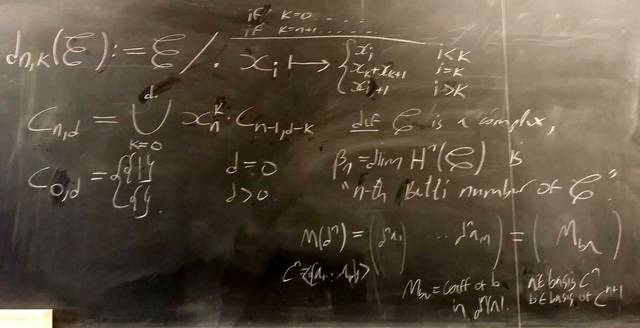171020-123521: Basic homology (3).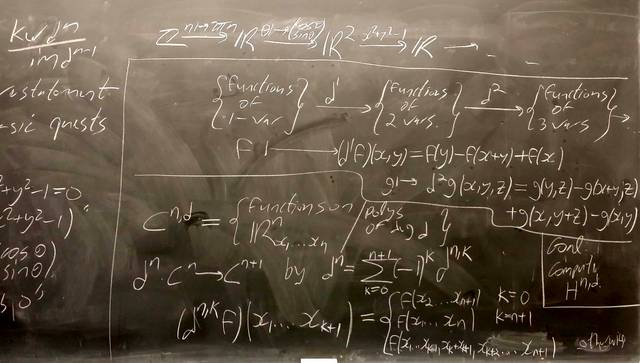171020-123520: Basic homology (2).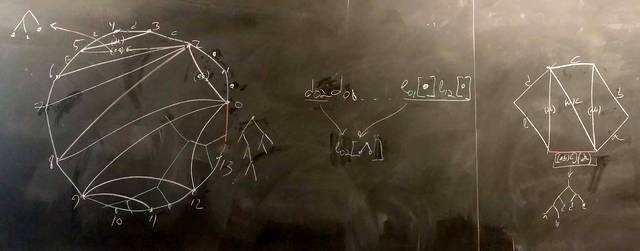171020-123518: From triangulations to trees.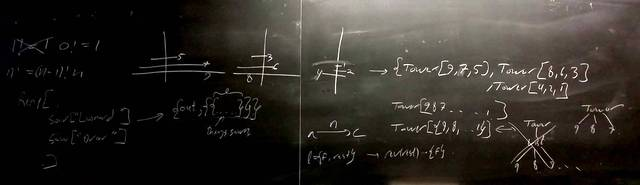171016-111042: The Towers of Hanoi (2).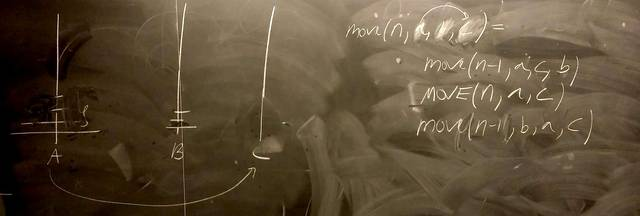171013-121553: The Towers of Hanoi.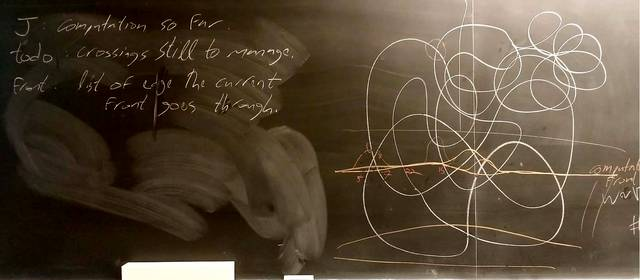171013-121552: Faster Jones computations (7).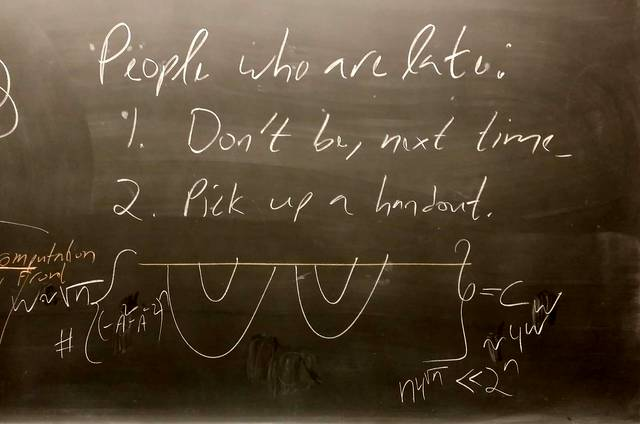171013-121551: Faster Jones computations (6).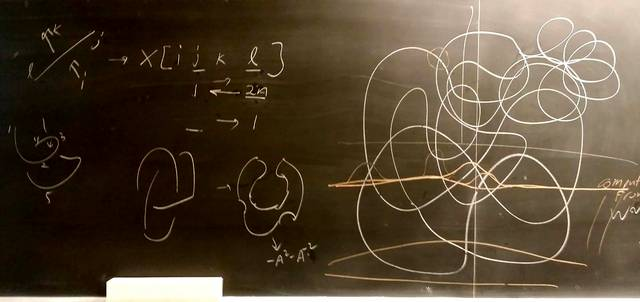171013-121550: Faster Jones computations (5).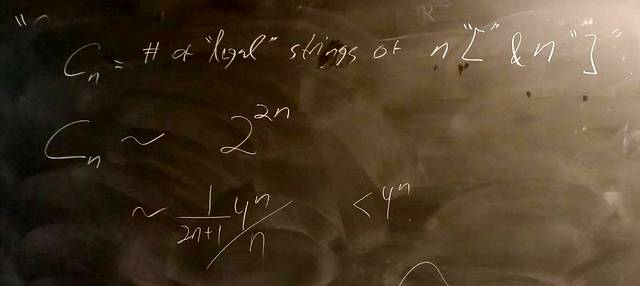171006-132436: Faster Jones computations (4).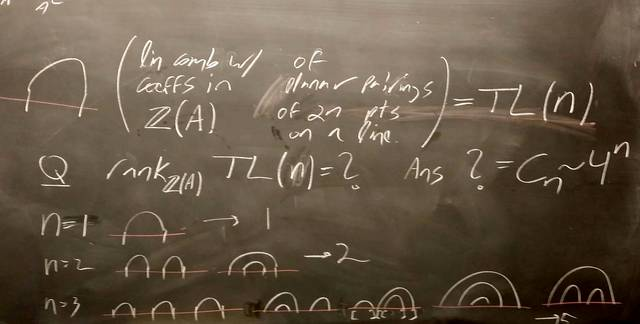171006-132435: Faster Jones computations (3).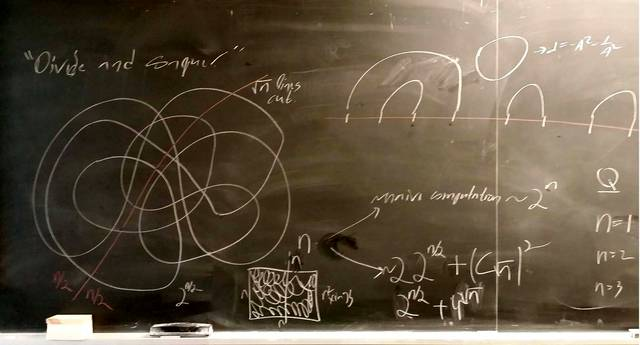171006-132434: Faster Jones computations (2).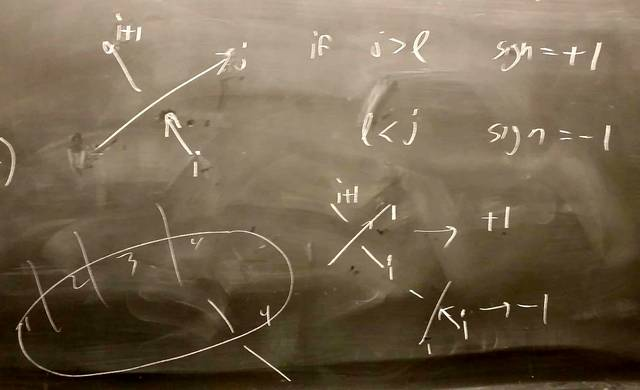171006-132433: Faster Jones computations.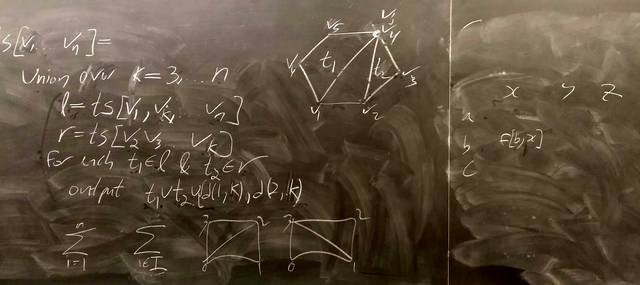170929-142836: Triangulations and the Catlan numbers (5).170929-142835: Triangulations and the Catlan numbers (4).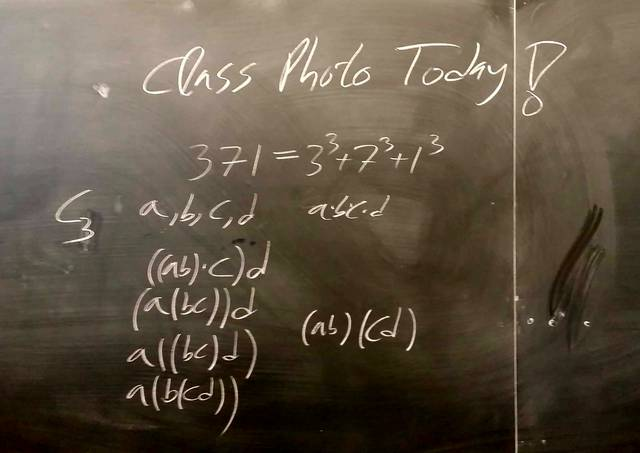170929-142834: Triangulations and the Catlan numbers (3).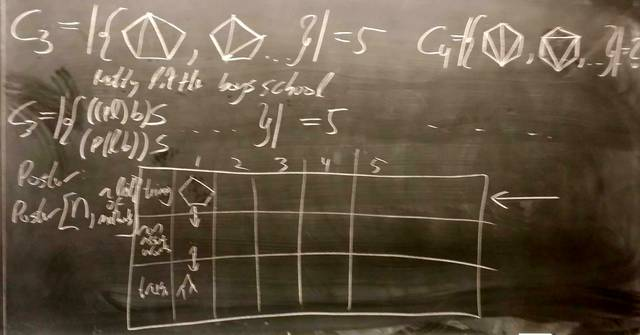170929-142833: Triangulations and the Catlan numbers (2).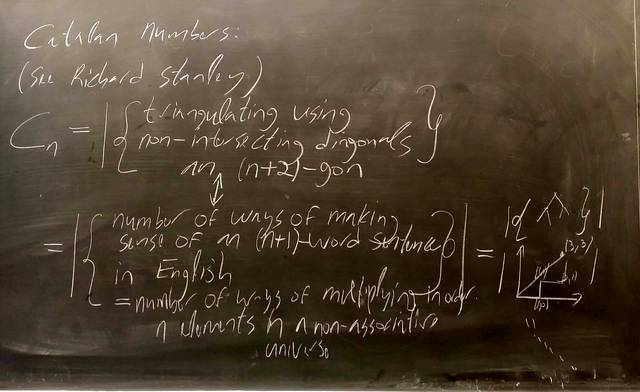170929-142832: Triangulations and the Catlan numbers.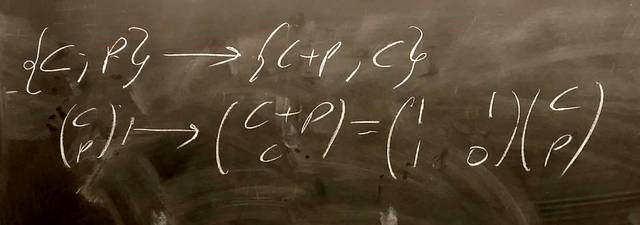170925-111213: Too many ways to compute the Fibonacci numbers (6).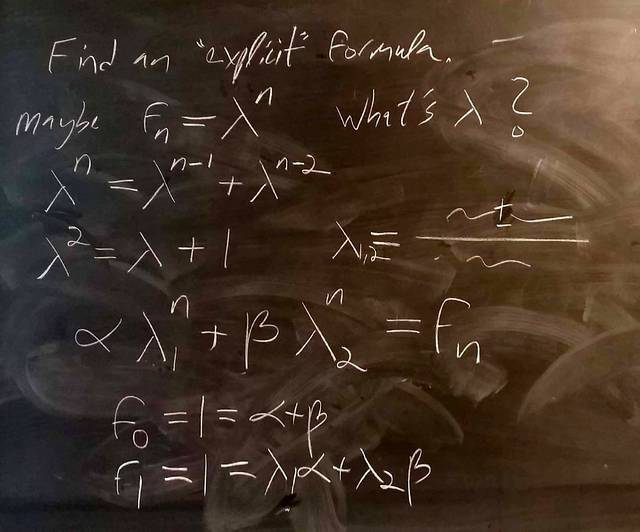170925-111212: Too many ways to compute the Fibonacci numbers (5).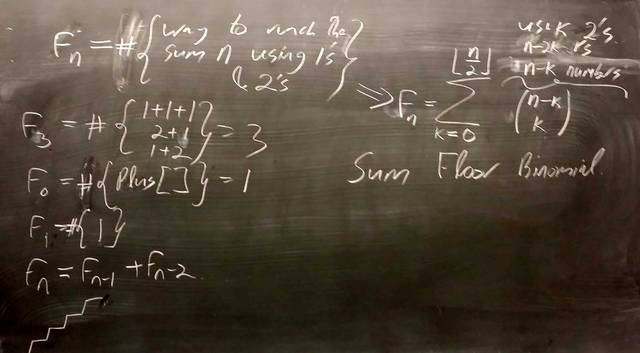170925-111211: Too many ways to compute the Fibonacci numbers (4).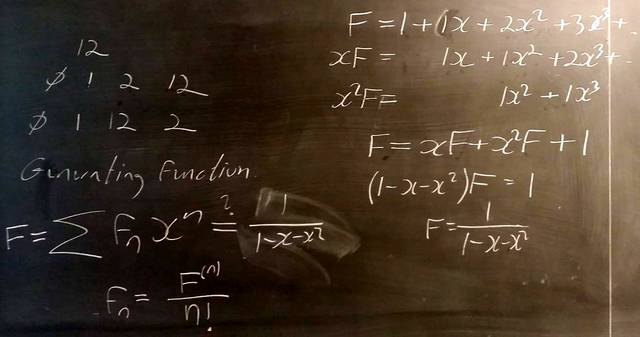170925-111210: Too many ways to compute the Fibonacci numbers (3).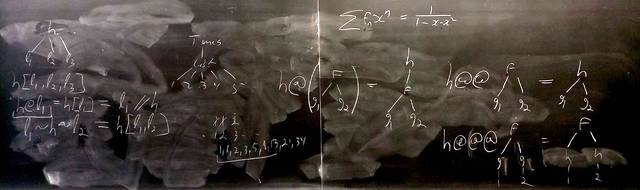170922-121309: Too many ways to compute the Fibonacci numbers (2).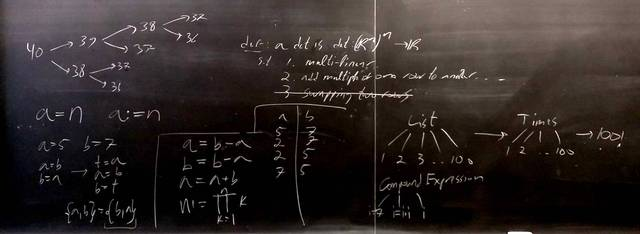170922-121308: Too many ways to compute the Fibonacci numbers.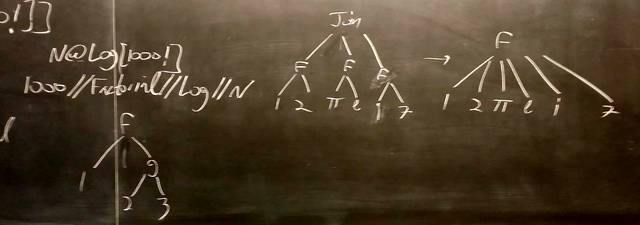170918-111027: Expressions in Mathematica (2).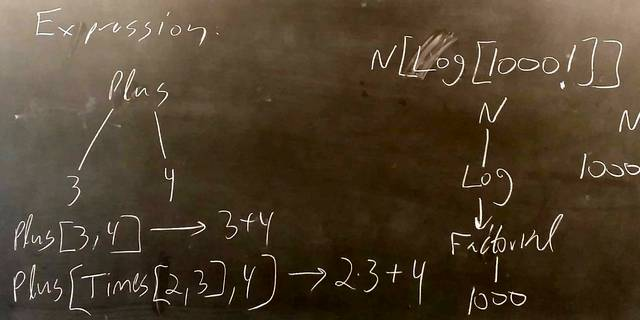170918-111026: Expressions in Mathematica.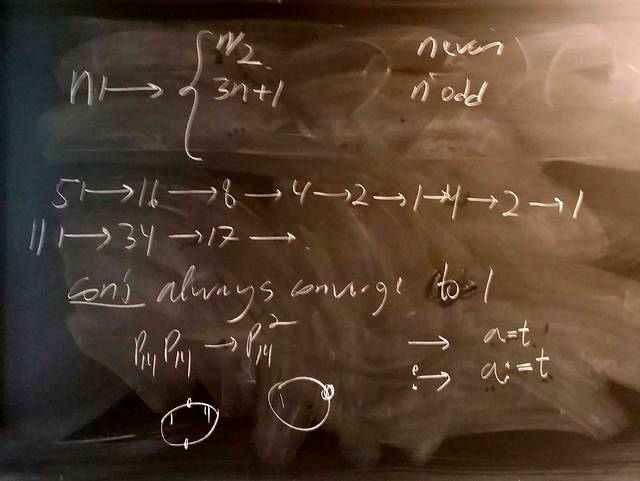170915-123319: The Collatz problem.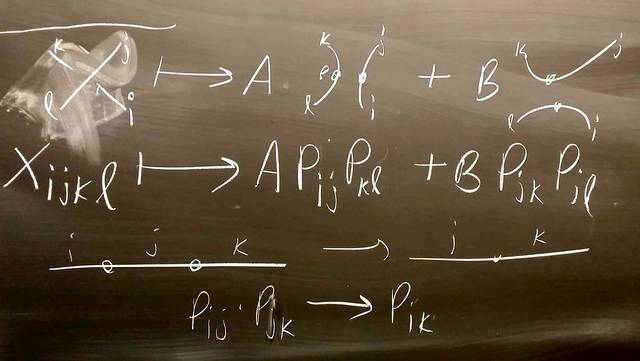170915-123318: Smoothings in Mathematica.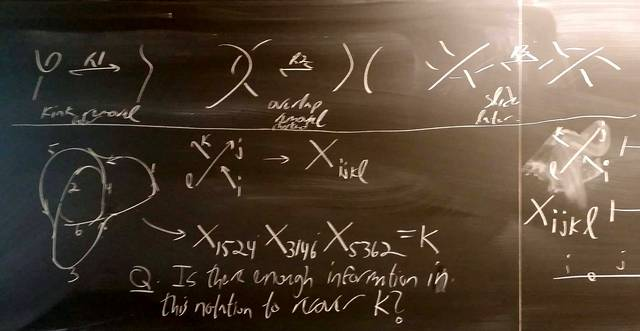170915-123317: Notation for planar diagrams.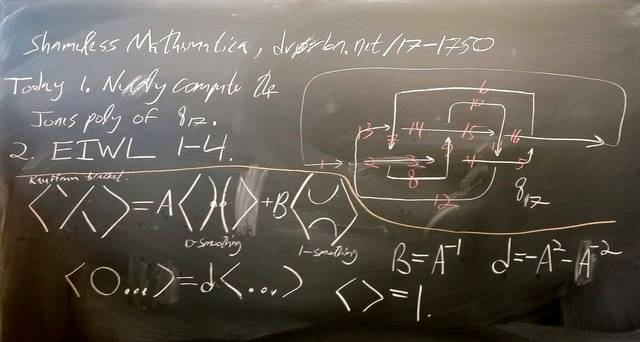170915-123316: Initial blackboard (2).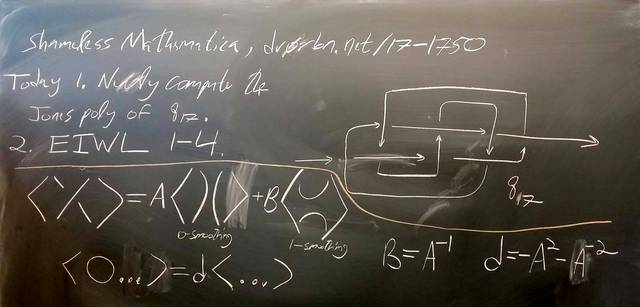170915-123315: Initial blackboard.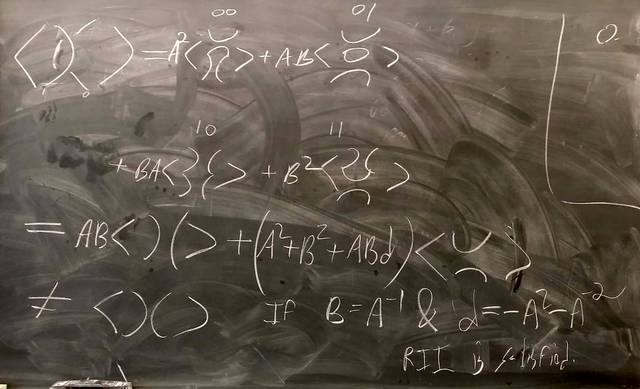170911-111546: The Kauffman bracket.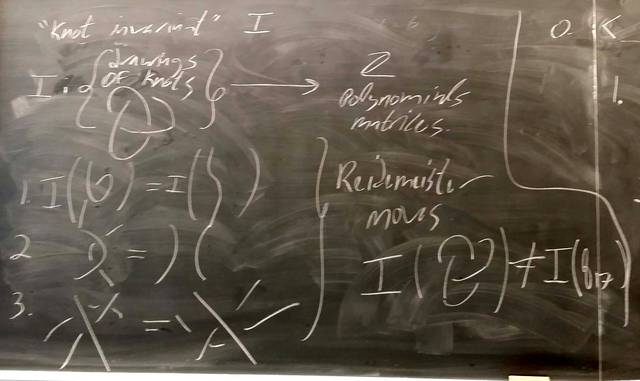170911-111545: Knot invariants and Reidemeister moves.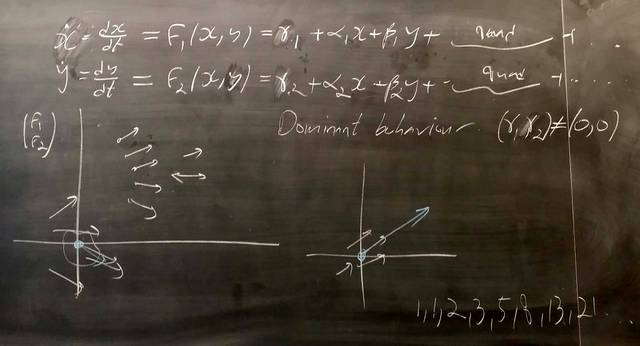170911-111543: ODEs and vector fields.